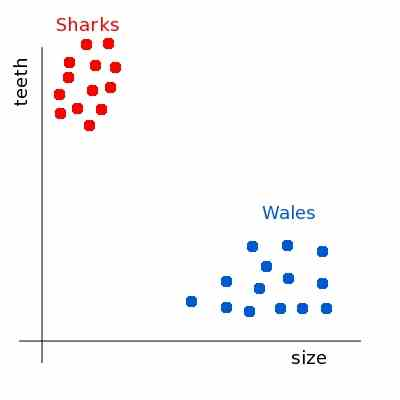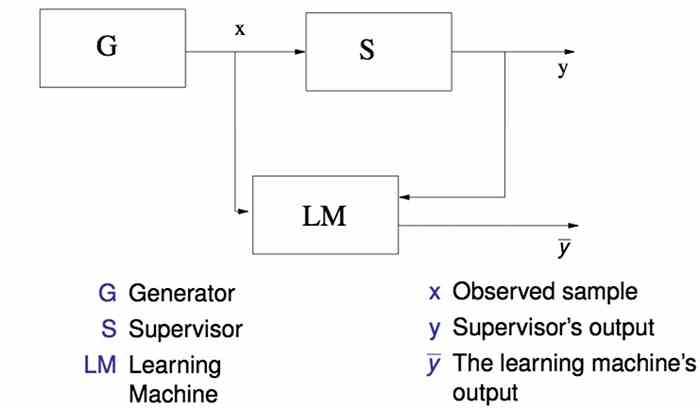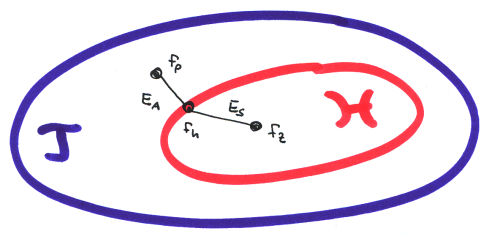## Machine Learning

Machine Learning was the core topic of my master thesis. For detailed information about the thesis take a look at the "projects" section. I decided to give a small introduction into machine learning for those who are not familiar with the topic.

At the beginning an example. Given two different classes of fishes (wales and sharks). These fishes differentiate in example in size and in their teeth. If we transform this into an 2D vector space, one axis represent the size, the other the teeth. Because of their different appearances the wales and sharks are arranged in a different area of the space:In the machine learning all events are discrete (you can not observe all wales and sharks of the world). The aim of machine learning is to find a function or an approximation of a function which describes a true predection given an "mostly" unknown distribution (in our case a function which is learned by some samples of wales and sharks and classifies a new one correctly). We take a look at a more abstract visualization:

The general learning model:A Generator generates some samples x. We have a supervisor which knows the true mapping function fp. The learning machine LM gets x and the supervisors output y. Now LM learns a function which predicts in most cases the same output as the supervisor (or in other words, it wants to minimize the false predictions). Now the further question, what is fp and how it can be found?T describes the target space, the among of all functions including the true function fp used by the supervisor. In most cases fp is unknown. Based on the samples we want to learn a function that imitates fp. Because of the unknown fp we choose a sub space of T called H with a finite set of functions. fn would be the best function in H that we could choose for approximating fp. Because of only a finite number of samples, fz is the best approximation that we can find (number of complexity equal to number of samples). EA is called the approximation error, ES is called the sample error. EA + ES is the true error between fp and fz. Wrong prediction may cause costs. Because of this our aim is to minimize the costs. We use the risk minimization principle for finding the function fz which reduces the sample error. The important questions is to find a good sub space H which is not too complex on the one hand, but powerful enough to represent a good fz on the other hand. The general rule is to keep the function a simple as it can be. If we look at a simple example. If we have two points in an 2 dimensional space. We can easy separate these two points linear with a line between them. Also with 3 points we can separate them easy in 2D. But if we have four points, we can not simple separate them in 2D, but if we transform them into 3D we can easy separate them linear. So number of samples-1 is the maximal complexity of H. This is called the VC dimension. So the algorithm starts with the simplest function and converge against the VC dimension by minimizing the risk.

This is only an abstract and small introduction into the general machine learning problem and how to solve it. A deeper view can be found in Pattern Recognition and Machine Learning (Information Science and Statistics) by Christopher M. Bishop.

### Search

Free Onepage Joomla! template by L.THEME